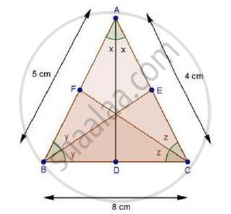Share

D, E and F Are the Points on Sides Bc, Ca and Ab Respectively of δAbc Such that Ad Bisects ∠A, Be Bisects ∠B and Cf Bisects ∠C. If Ab = 5 Cm, Bc = 8 Cm and Ca = 4 Cm, Determine Ap, Ce and Bd. - CBSE Class 10 - Mathematics

Question

D, E and F are the points on sides BC, CA and AB respectively of ΔABC such that AD bisects ∠A, BE bisects ∠B and CF bisects ∠C. If AB = 5 cm, BC = 8 cm and CA = 4 cm, determine AP, CE and BD.

SolutionIn ΔABC, CF bisects ∠C.

We know that, the internal bisector of an angle of a triangle divides the opposite side internally in the ratio of the sides containing the angle.

therefore"AF"/"FB"="AC"/"BC"

rArr"AF"/(5-"AF")=4/8       [∵ FB = AB – AF = 5 – AF]

rArr"AF"/(5-"AF")=1/2

⇒ 2AF = 5 – AF

⇒ 2AF + AF = 5

⇒ 3AF = 5

rArr"AF"=5/3 cm

Again, In ΔABC, BE bisects ∠B.

therefore"AE"/"EC"="AB"/"BC"

rArr(4-"CE")/"CE"=5/8     [∵ AE = AC – CE = 4 – CE]

⇒ 8(4 − 𝐶𝐸) = 5 × CE

⇒ 32 – 8CE = 5CE

⇒ 32 = 13CE

rArr"CE"=32/13 cm

Similarly,

"BD"/"DC"="AD"/"AC"

rArr"BD"/(8-"BD")=5/4        [∵ DC = BC – BD = 8 – BD]

⇒ 4BD = 40 – 5BD

⇒ 9BD = 40

rArr"BD"=40/9 cm

Hence, AF=5/3 cm, "CE"=32/13 cm and "BD"=40/9 cm.

Is there an error in this question or solution?

Video TutorialsVIEW ALL 

Solution D, E and F Are the Points on Sides Bc, Ca and Ab Respectively of δAbc Such that Ad Bisects ∠A, Be Bisects ∠B and Cf Bisects ∠C. If Ab = 5 Cm, Bc = 8 Cm and Ca = 4 Cm, Determine Ap, Ce and Bd. Concept: Angle Bisector.
S